Al-Khwarizmi

by Shawn Overbay, Jimmy Schorer, and Heather Conger

History

Al-Khwarizmi wrote numerous books that played important roles in arithematic and algebra. In his work, De numero indorum (Concerning the Hindu Art of Reckoning), it was based presumably on an Arabic translation of Brahmagupta where he gave a full account of the Hindu numerals which was the first to expound the system with its digits 0,1,2,3,....,9 and decimal place value which was a fairly recent arrival from India. Because of this book with the Latin translations made a false inquiry that our system of numeration is arabic in origin. The new notation came to be known as that of al-Khwarizmi, or more carelessly, algorismi; ultimately the scheme of numeration making use of the Hindu numerals came to be called simply algorism or algorithm, a word that, originally derived from the name al-Khwarizmi, now means, more generally, any peculiar rule of procedure or operation. The Hindu numerals like much new mathematics were not welcomed by all. In 1299 there was a law in the commercial center of Florence forbidding their use; to this day this law is respected when we write the amount on a check in longhand (ernie.bgsu.edu).

It was at the House of Wisdom that al-Khwarizmi wrote his treatise al-Kitab al-mukhtasar fi hisab al-jabr wa'l-muqabala or "The Compendious Book on Calculation By Completion and Balancing" that was written in 830 B.C.. It dealt with "what is easiest and most useful" (ernie.bgsu.edu). Conceived as an elementary textbook of practical mathematics, the Al-jabr wa'l-muqabala began with a discussion of the algebra of first and second degree equations and moved on in its final two parts to the business of practical applications to questions on mensuration and legacies. This was the most important book al-Khwarizmi was known for.

Al-jabr

From his most important book, Al-jabr wa'l muqabalah , comes the word algebra. The word al-jabr presumably meant something like "restoration" or "completion" and seems to refer to the transposition of subtracted terms to the other side of an equation; the word muqabalah is said to refer to "reduction" or "balancing"--that is, the cancellation of like terms on opposite sides of the equations or the simplification of the resulting expression (Boyer,228).

Diophantus is sometimes called "the father of Algebra," but this title more appropriately belongs to al-Khwarizmi. Al-Khwarizmi's work is on a more elementary and rhetorical level than that of Diophantus. Also, Arab scholars did not make any use of syncopation or of negative numbers. Al-jabr comes closer to elementary algebra of today than the works of either Diophantus or Brahmagupta, because the book is not concerned with difficult problems in indeterminant analysis but with a straight forward and elementary exposition of the solution of equations, especially that of second degree (Boyer, 228).

The Arabs loved good clear arguments from beginning to end as well as systematic organizations (which Diophantus of the Hindus excelled). The Arabs were more practical-minded and down-to-earth in their approach to mathematics (Boyer, 227-232).

In the Latin translation of al-Khwarizmi's Algebra , it opens with a brief introductory statement of the positional principle for numbers and then proceeds to the solution in six short chapters of six types of quadratics: (1) squares equal to roots, (=square root of 2), (2) squares equal to numbers, (=2), (3) roots equal to numbers (square root of x = 2), (4) squares and roots equal to numbers (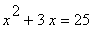), (5) squares and numbers equal to roots (), and (6) roots and numbers equal to squares () (members.aol.com). Chapter I covers the case of squares equal to roots, expressed in modern notation as,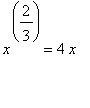,giving the answers x = 5, x = 12, and x= 2 respectively. Chapter II covers the case of squares equal to numbers and Chapter III solves the case of roots equal to numbers, with there illustrations per chapter to cover the cases in which the coefficient of the variable term is equal to , more than, or less than one. The other three chapters are more interesting and covers the three classical cases of three-term quadratic equations: (1)squares and roots equal to numbers, (2)squares and numbers equal to roots, and (3) roots and numbers equal to squares. Chapter IV includes the three illustrations,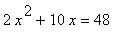,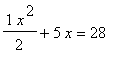. In each of these cases only the positive answers are given. In Chapter V only one example is used,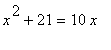, but both roots, 3 and 7. In Chapter VI only a single example agin is used,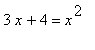and if the coefficient of the exponent is not unity, al-Khwarizmi reminds one to divide first by the coefficient. Once again the steps in completing the square are indicated and again only the positive root is given (Boyer, 229).

As can be seen quadratics were al-Khwarizmi's most important contribution to the mathematical world so we will show you an example of a quadratic graphed.

> with (plots):

> animate(j*k^2,k=-4..4,j=-4..4,frames=26,color=magenta);Origin of Algebra

It is the question of what type of inspriations inspired Arabic algebra? Judging from evidence internal to al-Khwarizmi's Al-jabr wa'l-muqabala , the mathematical environment in which his ideas developed included facts, theories, and approaches from several recognizable sources. It is stated that no categorical answer can be given; but the arbitrariness of the rules and the strictly numerical form of the six chapters carries an ancient Babylonian and medieval Indian mathematics. Also, Mesopotamia is more likely a source than India in the prgression of Arabic mathematics because of the exclusion of indeterminant analysis and the avoidance of syncopation. Therfore, there are three main schools of thought on the origin of Arabic algebra: Hindu influences, Mesopotamia, and Greek inspiration (Boyer,227-232).

Also, Arabic algebra had much in common with Greek geometry although the first part of the book or arithmetic resembles no part of Greek mathematics. Al-Khwarizmi's use of geometrical justifications of algebraic manipulations together with the fact that the Elements existed in two distinct translations from Greek into Arabic by his contemporary at the House of Wisdom, al-Hajjaj ibn Yusuf ibn Matar, suggest a line of descent from Euclid. On the other hand, because his treatment of practical geometry so closely followed that of the Hebrew text, Mishnat ha Middot, which dated from around 150 A.D., the evidence of Semitic ancestry exists. Al-Khwarizmi also gives geometric proofs of these methods (lib.virginia.edu).

Geometric Foundation

Al-Khwarizmi used geometric proofs when solving the six types of quadratic equations he used in his book Al-jabr. We will be proving the equations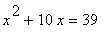andfrom the book. First, we will be drawing the figure in which al-Khwarizmi used in order to prove these equations. The proof for equation.

> with(plots):

> l1:=n->[seq([5,i],i=0..n)]:

`Warning, `i` in call to `seq` is not local`

> l2:=n->[seq([i,1],i=0..n)]:

`Warning, `i` in call to `seq` is not local`

> l3:=n->[seq([1,i],i=0..n)]:

`Warning, `i` in call to `seq` is not local`

> l4:=n->[seq([i,5],i=0..n)]:

`Warning, `i` in call to `seq` is not local`

> l5:=n->[seq([6,i],i=0..n)]:

`Warning, `i` in call to `seq` is not local`

> l6:=n->[seq([i,0],i=0..n)]:

`Warning, `i` in call to `seq` is not local`

> l7:=n->[seq([0,i],i=0..n)]:

`Warning, `i` in call to `seq` is not local`

> l8:=n->[seq([i,6],i=0..n)]:

`Warning, `i` in call to `seq` is not local`

> display([polygonplot(l1(6)),polygonplot(l2(6)),polygonplot(l3(6)),polygonplot(l4(6)),polygonplot(l5(6)),polygonplot(l6(6)),polygonplot(l7(6)),polygonplot(l8(6)),textplot([.5,3,'f']),textplot([3,.5,'e']),textplot([3,5.5,'c']),textplot([5.5,3,'d']),textplot([1.5,4.5,'a']),textplot([4.5,1.5,'b'])],axes=none);Al-Khwarizmi drew a square ab to represent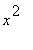, and on the four sids of this square he placed rectangles c , d , e , and f , each 2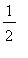units wide. To complete the larger square, one must add the four small corner squares each of which has an area of 6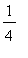units. hence, to "complete the square" we add 4 times 6units or 25 units, thus obtaining a square of total area 39 + 25 = 64 units (as is clear from the right-hand side of the given equation). The side of the large square must, therefore, be 8 units, from which we subtract 2 times 2, or 5, units to find that x = 3, thus proving that the answer found in Chapter IV is correct.

Now, proving the equationwith geometry. First with a figure of this proof:

> s1:=n->[seq([i,2],i=0..n)]:

`Warning, `i` in call to `seq` is not local`

> s2:=n->[seq([i,5],i=0..n)]:

`Warning, `i` in call to `seq` is not local`

> s3:=n->[seq([0,i],i=2..n)]:

`Warning, `i` in call to `seq` is not local`

> s4:=n->[seq([10,i],i=2..n)]:

`Warning, `i` in call to `seq` is not local`

> s5:=n->[seq([3,i],i=2..n)]:

`Warning, `i` in call to `seq` is not local`

> s6:=n->[seq([5,i],i=0..n)]:

`Warning, `i` in call to `seq` is not local`

> s7:=n->[seq([i,0],i=5..n)]:

`Warning, `i` in call to `seq` is not local`

> s8:=n->[seq([7,i],i=0..n)]:

`Warning, `i` in call to `seq` is not local`

> s9:=n->[seq([10,i],i=0..n)]:

`Warning, `i` in call to `seq` is not local`

> display([polygonplot(s1(10)),polygonplot(s2(10)),polygonplot(s3(5)),polygonplot(s4(5)),polygonplot(s5(5)),polygonplot(s6(5)),polygonplot(s7(10)),polygonplot(s8(2)),polygonplot(s9(2)),textplot([0,5.25,'a']),textplot([5,5.25,'t']),textplot([10,5.25,'g']),textplot([0,1.5,'h']),textplot([3,1.5,'b']),textplot([5.35,1.5,'e']),textplot([7.35,1.5,'n']),textplot([10.35,2,'d']),textplot([5,-.5,'c']),textplot([7,-.5,'m']),textplot([10,-.5,'l'])],axes=none,scaling=constrained);The geometric proofs for Chapters V and VI are somewhat more complicated. First the square ab representsand the rectangle bg represents 21 units. Then the large rectangle, comprising the square and the rectangle bg , must have an area equal to, so that the side ag or hd must be 10 units. If then, one bisects hd at e , draws et perpendicular to hd , extends te to c so that tc = tg , and completes the squares tclg and cmne , the area tb is equal to area md . But tl is 25, and the gnomon tenmlg is 21 (since the gnomon is equal to the rectangle bg ). Hence, the square nc is 4, and its side ec is 2. Inasmuch as ec = be , and since he = 5, we see that x = hb = 5 - 2 or 3, which proves that the arithmetic solution given in Chapter V is correct (Boyer, 231-232).

There is also a link in which you can visit where this demonstration is first wrote in Arabic and then with a change of a letter you can see it translated into American. These are the links: http://www-history.mcs.st-and.ac.uk/~history/Bookpages/Al'Khwarizmi4c.gifhttp://www-history.mcs.st-and.ac.uk/~history/Bookpages/Al'Khwarizmi4b.gif

Other Works of al-Khwarizmi

Al-Khwarizmi is also responsible for developing trigonometric tables containing sine functions. They were later used to help form tangent functions. He also developed the calculus of two errors, which led him to the concept of differentiation. He was also an astronomer as mentioned previously, and he wrote a treatise on astronomy, and also a book on astronomical tables. It was not until his books on astronomy were translated into Latin that the West was introduced to these new scientific concepts. His work with geography also contributed a great deal to society. Al-Khwarizmi revised Ptolemy's views on geography. He had seventy geographers working under his leadership, and they produced the first map of the known world in 830 B.E.. Al-Khwarizmi also was the first to attempt to get a measurement of the volume and circumference of the earth. His book on geography was entitled "Kitab surat-al-Ard," which included maps. Al-Khwarizmi also did much work relative to time. He wrote works on clocks, astrolabes, and sundails (erols.com).

Modern Day Problems of Al-Khwarizmi

Here is a problem which al-Khwarizmi solved in his book Al-jabr set in modern tones.

Jill buys 2 bars of Snickers at \$ X.00 each and 4 bars of Mars at \$Y.00 each. She pays \$14.00 for the lot. Jack buys 1 bar of Snickers and manages to sell 3 bars of Mars each to the shopkeeper. He ends up making \$8.00 for himself. This translates into the simultaneous equations 2x + 4y=14 and x - 3y = -8. How much do Snickers and Mars cost?

You can solve this problem by substitution.

> Q:=2*x+4*y=14;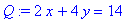> P:=x-3*y=-8;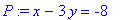> x=3*y-8;> Q;> solve (Q);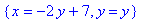> y=3;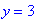> evalf (3*(3)-8);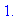Therefore Snickers cost \$1.00 and Mars cost \$3.00 (dmu.ac.uk).

Another problem that al-Khwarizmi would have worked on, also set in modern times, is one dealing with the formation of equations to determine force using an equation for velocity in terms of time, t. This problem also uses differentiation which al-Khwarizmi also helped discover.

The velocity of the bullet is V and its mass is 0.01kg. Superman needs to work out the force acting on the bullet when he blows on it at time 0.1 seconds, and blow just hard enough to stop it without blowing it back to Lois. V=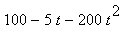Now, force = mass x acceleration

Mass is given 0.01 kg and acceleration can be found by differentiating velocity.

> A:=dV/dt=-5-400*t;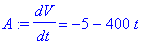Therefore force

F=.01 x {-5-400t}...t = 0.1

> F:=.01*(-5-400*(.1));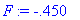So the force of air resistance acting on the bullet is 0.45 N opposite to the direction of travel. Superman needs to add to this resistance just enough to equal the velocity and hence stop the bullet.

V = F - G

> 100-5*t-200*t^2=-.45-G,t=.1;> -97.5=-.45-G;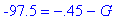> G:=52.2,N;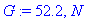>

So superman will have to muster up exactly 52.5 N of wind force to halt the bullet (dmu.ac.uk).

Conclusion

As can be seen al-Khwarizmi was a noteable mathematician along with an abundance of other attributes. He discovered new ways of solving quadratic equations with algebra while keeping the problems simple and easy to manipulate. Al-Khwarizmi's ways of working with quadratic equations were so popular that his book Al-Jabr was used as the principle mathematics book at European universities until the 16th century (erols.com).

Biblography

(1) http://ernie.bgsu.edu/~vrickey/math311/al-khwarizmi.txt. "Abu Ja'far Muhammed ibn Al-Khwarizmi."

(2)http://www.lib.virginia.edu/science/parshall/algebra.html#ARAB. "Algebra in the Arab World: Al-Khwarizmi, Abu-Kamil, and Al-Karaji."

(4)Boyer, Carl B. History of Mathematics . John Wiley & Sons, Inc. Canada. pp 227-232.

(5)http://members.aol.com/bbyars1/algebra.html. "Al'Khwarizmi & Al Jabr."

(8)http://www.erols.com/zenithco/Khwariz.html."Al-Khwarizmi."

(9)http://www.dmu.ac.uk/ln/library/teams/lois.html. "Lois Pulls The Trigger."